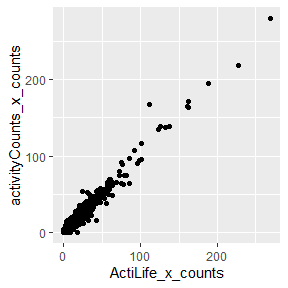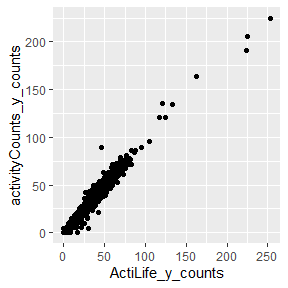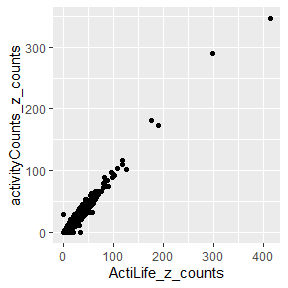# Introduction

Many research areas use accelerometers to study people’s activities including sleep, sedentary behaviour, and physical activity. Actigraph is one of the most common research grade accelerometers. Actigraph accelerometers include ActiLife is a closed-source software, which generates activity counts. There is considerable research validating and developing algorithms for human activity using Actilife counts. Unfortunately, Actilife counts are proprietary and difficult to implement if researchers use different accelerometer brands. Recently, Brond et al. developed a code in MATLAB, which can convert raw accelerometer data to Actilife counts. Their work can help researchers use different accelerometers and calculate Actilife counts, MATLAB is a commercial program. Unlike MATLAB, R is open-source, and also R is very popular among health and activity researchers. The package activityCounts allow users to convert the accelerometer data to Actilife counts.

# How to use

## Install R

Visit R Project to download and install R.

## Install activityCounts

You can install the released version of activityCounts from CRAN with running the following code in the R console:

install.packages("activityCounts")

And the development version from GitHub with:

install.packages("devtools")
devtools::install_github("walkabillylab/activityCounts")

## Load activityCounts

To load the package and its sample datasets run the following in R console:

library(activityCounts)

## Import your data

To generate the counts, import your data from your source file. Store the data inside a variable so you can pass that the main function of the package, counts(). For example, you can use the read_csv() function from the tidyverse package to import your data: Note that the dataset must have three columns which X, Y, and Z readings.

# install.packages("tidyverse")
raw_accel_data <- tidyverse::read_csv("~/your_raw_accelerometer_data.csv")

## Use the counts() function

To generate the counts, provide the function counts() with your data and the sampling frequency of the data.

# assume sampling frequency is 100 Hz
sampling_frequency <- 100
counts_measures <- activityCounts::counts(data = raw_accel_data, hertz = sampling_frequency)

Now the variable counts_measures contains three columns which indicate the calculated counts for X, Y, and Z axes respectively. If the data frequency is less than 30 Hz, first resample your data and increase the frequency to more than 30 Hz and then calculate the counts.

# Example

## Check the sample input dataset:

activityCounts package includes a sample dataset which is available for testing by importing the package. The name of the dataset is sampleXYZ and its sampling frequency is 100Hz. To check out sampleXYZ:

data("sampleXYZ")
head(sampleXYZ)
#>                  Time accelerometer_X accelerometer_Y accelerometer_Z
#> 1 2019-01-01 00:00:00          -0.035           0.816          -0.063
#> 2 2019-01-01 00:00:00          -0.047           0.805          -0.023
#> 3 2019-01-01 00:00:00          -0.031           0.793          -0.008
#> 4 2019-01-01 00:00:00          -0.020           0.809           0.012
#> 5 2019-01-01 00:00:00          -0.016           0.844           0.023
#> 6 2019-01-01 00:00:00          -0.004           0.879           0.012

## How to calculates counts:

For sampleXYZ data, the first column is X, second is Y, and the third column is the Z axis, therefore when calling the function, specify the columns in the argument section. Now by applying the function counts() calculate the counts for sampleXYZ.

sampleXYZ_counts <- counts(data = sampleXYZ, hertz = 100 ,x_axis = 1,y_axis = 2,z_axis = 3)
head(sampleXYZ_counts)

### Use your own data:

By default, the second column of your dataset should contain accelerometer data for x-axis, the third column for y-axis and the forth column for the z-axis However, you can specifically tell the counts() function which columns have the x, y, and z-axis data. Use x_axis, y_axis, and z_axis arguments when calling the function to specify the desired columns for each axes.

In this example code, the first column has the time stamp for the data. The second column has the x-axis data, the third column has the y-axis data, and the fourth column has the z-axis data. Therefore, assuming the sampling frequency is 100 Hz, we call the function like this:

calculated_output <- counts(data = your_raw_data, hertz = 100, x_axis = 2, y_axis = 3, z_axis = 4)

The default values for x_axis, y_axis, and z_axis are one, two, and three respectively. So if you don’t specify them, the function assumes the first column is for the x-axis, the second for the y-axis and the third is for the z-axis.

## Compare the sample outputs:

The other dataset that comes with this package has nine columns; the first three columns are the raw accelerometer data from sampleXYZ, the next three columns are the counts calculated by the counts() function for X, Y, and Z axes. Finally, the last three columns are counts calculated by the ActiLife software. To check the data run the following:

summary(sampleCounts)
#>       Time                     activityCounts_x_counts
#>  Min.   :2019-01-01 00:00:00   Min.   :  0.0
#>  1st Qu.:2019-01-01 00:11:14   1st Qu.:  0.0
#>  Median :2019-01-01 00:22:29   Median :  0.0
#>  Mean   :2019-01-01 00:22:29   Mean   : 10.2
#>  3rd Qu.:2019-01-01 00:33:44   3rd Qu.: 19.0
#>  Max.   :2019-01-01 00:44:59   Max.   :280.0
#>  activityCounts_y_counts activityCounts_z_counts ActiLife_x_counts
#>  Min.   :  0.00          Min.   :  0.00          Min.   :  0.000
#>  1st Qu.:  0.00          1st Qu.:  0.00          1st Qu.:  0.000
#>  Median :  0.00          Median :  0.00          Median :  0.000
#>  Mean   : 16.33          Mean   : 12.51          Mean   :  9.849
#>  3rd Qu.: 37.00          3rd Qu.: 24.00          3rd Qu.: 18.000
#>  Max.   :225.00          Max.   :347.00          Max.   :269.000
#>  ActiLife_y_counts ActiLife_z_counts
#>  Min.   :  0.00    Min.   :  0.00
#>  1st Qu.:  0.00    1st Qu.:  0.00
#>  Median :  0.00    Median :  0.00
#>  Mean   : 16.48    Mean   : 13.87
#>  3rd Qu.: 37.00    3rd Qu.: 27.00
#>  Max.   :253.00    Max.   :413.00

## Visualize the difference:

As the summary shows, the counts calculated by activityCounts package and the software ActiLife are very close. In these plots, counts calculated by the package are sketched versus the counts calculated by the ActiLife software. The straight lines show strong correlations: